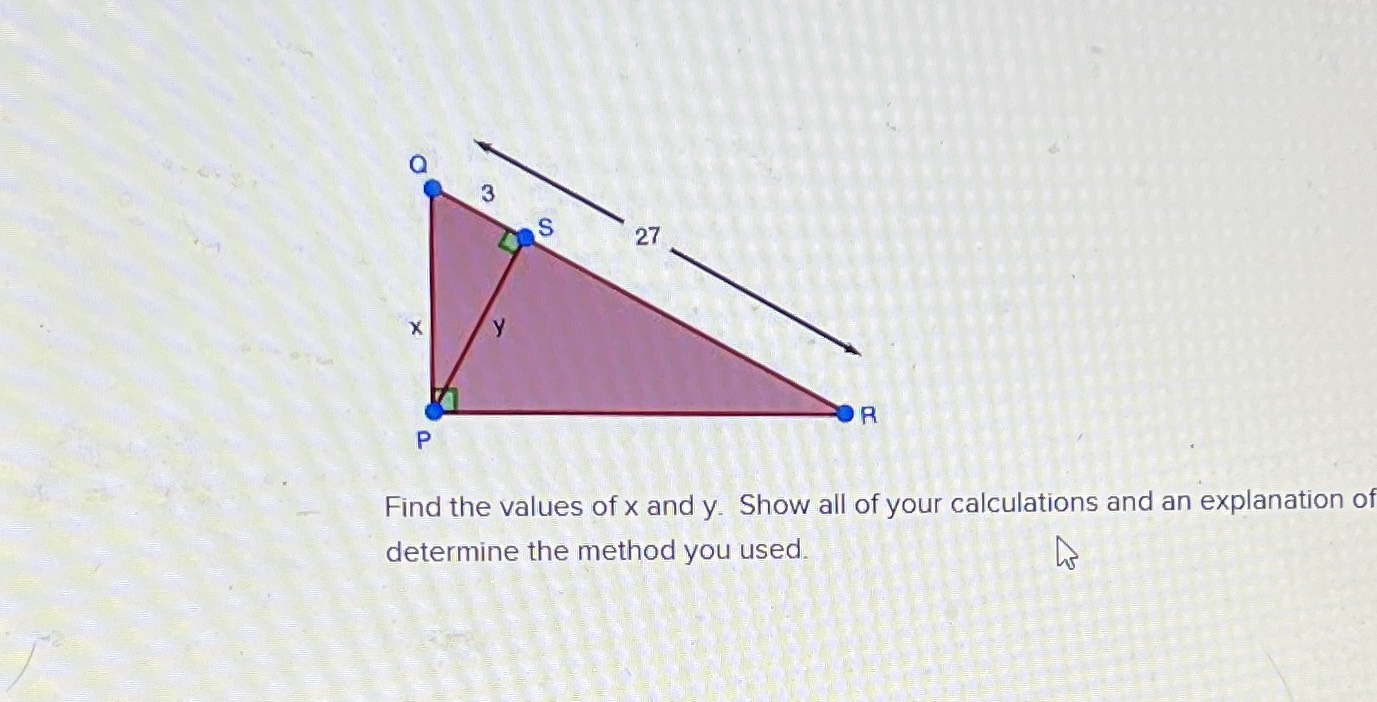### Still have math questions?

Trigonometry
QuestionFind the values of $$x$$ and $$y$$ . Show all of your calculations and an explanation of determine the method you used.

y=6$$\sqrt{2}$$